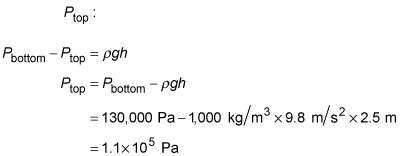##### Physics I: 501 Practice Problems For Dummies (+ Free Online Practice)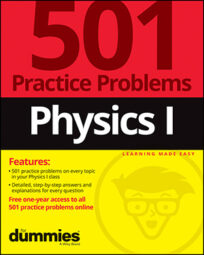Using physics, you can determine how pressure is affected by depth. For example, when swimming, you can calculate the change in water pressure when you change your diving depth.

Here are some practice questions that you can try.

## Practice questions

1. The pressure at the top of a pipe full of water is 101 pascals. What is the change in pressure between the top and the bottom of the pipe, 3.4 meters lower?

2. One end of a 50-meter-long hose is attached to the bottom of a large basin full of water. How many meters below the top of the basin must the hose outlet be positioned for the water pressure at the outlet to be 18,000 pascals?

3. While diving with a friend, you note that the water pressure at your current depth is 130,000 pascals. If you swim up another 2.5 meters, what is the water pressure?

The following are the answers to the practice questions:

1.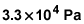The change in pressure is given by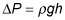In this case,and h = 3.4 meters. Insert these values into the equation: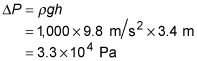2. 1.8 m

The change in pressure is given byIn this case,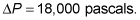Solve this equation for the height h: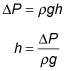Insert the known values into the equation: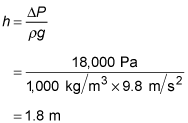3. 3.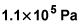The change in pressure is given byBy using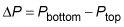the first equation becomes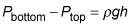You know that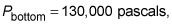so you can solve for